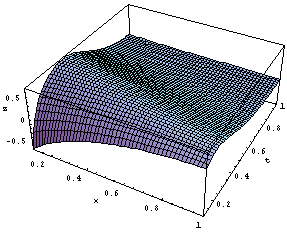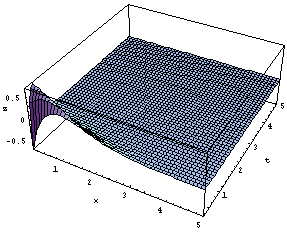Fig 1.  The surface graphics of the function u (x , t)  when  k = 6,  a = 1,  f(theta) = theta.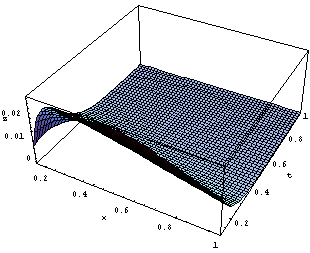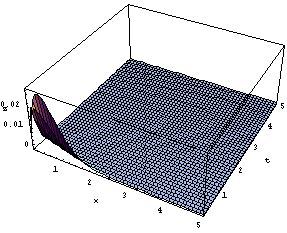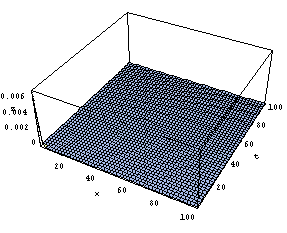Fig 2.  The surface graphics of the function v (x , t)  when  k = 6,  a = 1,  f(theta) = theta.